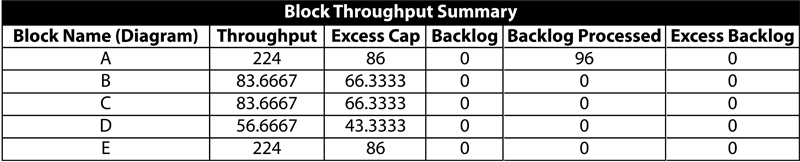# Phase Throughput Examples

These examples also appear in the System Analysis Reference book.

### Constant Throughput Example

To examine throughput in phases, consider a phase diagram of three phases with linked RBDs, as shown in the figure below. Each phase has a duration of 10 hours and all phases are set to continue simulation upon failure. Blocks A through E process a number of items per hour as identified next to each letter (e.g., A:10 implies that 10 units are processed per hour by block A). Similarly, each phase is marked with the constant throughput sent to the blocks in the phase (e.g., P1:5 implies phase P1 sends 5 units to its blocks ). For the sake of simplicity, it is assumed that the blocks can never fail and that items are routed equally to each path (see Throughput Analysis Options). The ignore backlog option is left unchecked for all blocks in the phase diagram.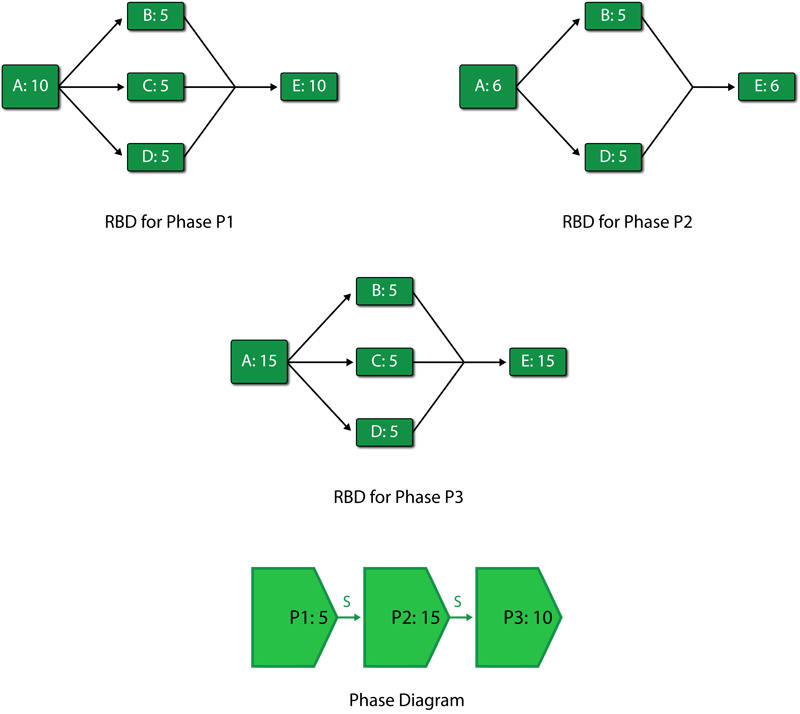If the phase diagram is run on a throughput simulation with an end time of 60 hours, the following scenario can be observed:

1. Phase P1 from 0 to 10 hours
a) Phase P1 sends 5 units in the first hour to block A. The capacity of block A in this phase is 10 units per hour. Block A processes these 5 units with an excess capacity of 5 units in the first hour.
b) The 5 units processed by block A are routed equally over the three paths to blocks B, C and D. Each of these blocks receives 1.67 units in the first hour. The capacity of each of these blocks is 5 units per hour. Thus, blocks B, C and D each process 1.67 units with an excess capacity of 3.33 units in the first hour.
c) Blocks B, C and D route each of their 1.67 units to E. The capacity of E in this phase is 10 units per hour. E processes the total of 5 units with an excess capacity of 5 units in the first hour.
d) The above steps are repeated for the first ten hours to complete phase P1.
A summary result table for phase P1 is shown next.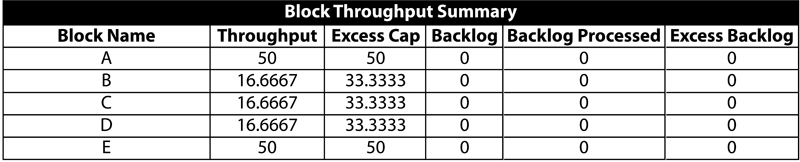2. Phase P2 from 10 to 20 hours
a) Phase P2 sends 15 units in its first hour to block A. The capacity of block A in this phase is changed from the previous phase and is now 6 units per hour. Thus, block A is able to process only 6 units while there is a backlog of 9 units per hour.
b) The 6 units processed by block A are routed equally to blocks B and D as C is absent in this phase. Blocks B and D get 3 units each. The capacity of each of these blocks is 5 units per hour. Thus, blocks B and D process 3 units with an excess capacity of 2 units each in the first hour of the second phase.
c) Blocks B and D route a total of 6 units to E. The capacity of block E in this phase is 6 units per hour. Thus, block E processes 6 units in the first hour of phase 2 with no excess capacity and no backlog.
d) The above steps are repeated for the ten hours of phase P2. At 20 hours phase P2 ends.
A summary result table for phase P2 is shown next.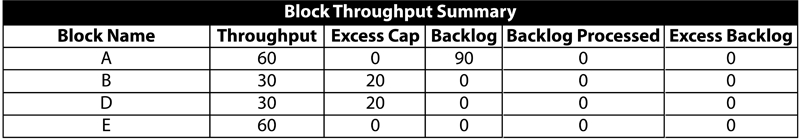3. Phase P3 from 20 to 30 hours
a) Phase P3 sends 10 units in its first hour to block A. The capacity of block A in this phase is now 15 units per hour. Thus, block A is able to process all 10 units sent by P3 and also process 5 units of the backlog from phase P2. Thus, during the first hour of phase P3, block A processes 15 units that includes a backlog of 5 units. Since block A is used to its full capacity, the excess capacity of A is zero.
b) The 15 units processed by block A in the first hour are routed equally over three paths to blocks B, C and D as block C is available again in this phase. Blocks B, C and D receive 5 units each. The capacity of each of these block is 5 units per hour. Thus, blocks B, C and D process 5 units each to full capacity with no excess capacity during the first hour of the third phase.
c) Blocks B, C and D route each of their 5 units to E. The capacity of block E in this phase is 15 units per hour. Thus, block E processes 15 units in the first hour of phase P3 with no excess capacity and no backlog.
d) The above steps are repeated for the ten hours of phase P3. It should be noted that although some of the backlog from phase P2 gets processed in phase P3 $\displaystyle (5\times 10=50\,\!$ units from the backlog get processed ), not all 90 backlogged units are processed ( $\displaystyle 90-50 = 40\,\!$ units remain). The remaining units cannot be shown as backlog for phase P3 because these were generated in phase P2. To avoid confusion between backlogs of different phases, BlockSim does not display backlog values at the individual phase levels. At 30 hours, phase P3 ends. This also ends the first cycle of the phase diagram.
A summary result table for phase P3 is shown next.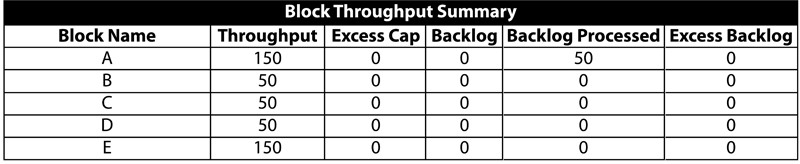4. Phase P1 in the second cycle from 30 to 40 hours
a) As in the first cycle, phase P1 sends 5 units to block A in the first hour. The capacity of block A in this phase is 10 units per hour. Thus, block A is able to process all 5 units sent by P1 and also process 5 units of the backlog from the first cycle. Thus, during the first hour of the second cycle of phase P1, block A processes 10 units that includes a backlog of 5 units. Since there is a backlog of 40 units from the first cycle, this is processed by block A in the first eight hours of the second cycle. During the last two hours of the second cycle of phase P1, block A processes only the 5 units per hour it receives from P1 and has an excess capacity of 5 units per hour. Thus, by the end of this phase, block A processes a total of 90 units (i.e., 50 units from P1 and 40 units of backlog) with an excess capacity of 10 units.
b) Units processed by block A are routed equally to blocks B, C and D. During the first eight hours of the second cycle of phase P1, 10 units per hour are sent by block A to blocks B, C and D. Each of these blocks gets 3.33 units per hour. The capacity of each of these blocks is 5 units per hour. Thus, during the first eight hours of P1 in the second cycle, blocks B, C and D process 3.33 units per hour with an excess capacity of 1.67 units per hour. During the last two hours, block A processes 5 units per hour. Thus, blocks B, C and D process 1.67 units per hour with an excess capacity of 3.33 units per hour. At the end of the phase, blocks B, C and D each process a total of 30 units $\displaystyle (3.33\times 8+1.67\times 2=30)\,\!$ with an excess capacity of 20 units $\displaystyle (1.67\times 8+3.33\times 2=20)\,\!$ .
c) Blocks B, C and D route each of their units to block E. The capacity of E in this phase is 10 units per hour. Block E processes 10 units per hour with no excess capacity during the first eight hours of the phase. During the last two hours, block E processes 5 units per hour with an excess capacity of 5 units per hour. By the end of the phase, block E processes a total of 90 units with an excess capacity of 10 units.
A summary result table for the second cycle of phase P1 is shown next.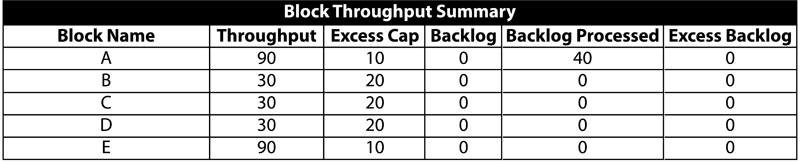5. Phase P2 in the second cycle from 40 to 50 hours
a) As in the first cycle, phase P2 sends 15 units in its first hour to block A. The capacity of block A in this phase is 6 units per hour. Thus, block A processes only 6 units while there is a backlog of 9 units per hour.
b) The 6 units processed by block A are routed equally to blocks B and D, as C is absent in this phase. Blocks B and D receive 3 units each. The capacity of each of these blocks is 5 units per hour. Thus, in the second cycle, blocks B and D process 3 units each with an excess capacity of 2 units each in the first hour of the second phase.
c) Blocks B and D route a total of 6 units to block E. The capacity of E in this phase is 6 units per hour. Thus, block E processes 6 units in the first hour of the phase with no excess capacity and no backlog.
d) The above steps are repeated for the ten hours of phase P2. At 50 hours, phase P2 ends in the second cycle.
A summary result table for the second cycle of phase P2 is shown next.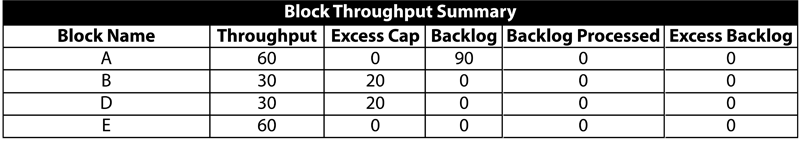6. Phase P3 in the second cycle from 50 to 60 hours
a) Phase P3 sends 10 units in its first hour to block A in the second cycle. The capacity of block A in this phase is 15 units per hour. Thus, block A is able to process all 10 units sent by P3 and also process 5 units of backlog from the second cycle of phase P2. Thus, during the first hour of phase P3, block A processes 15 units that includes a backlog of 5 units. Since block A is used to its full capacity, the excess capacity of A is zero.
b) The 15 units processed by block A in the first hour are routed equally over three paths to blocks B, C and D, as block C is available again in this phase. Blocks B, C and D receive 5 units each. The capacity of each of these block is 5 units per hour. Thus, blocks B, C and D process 5 units each to full capacity with no excess capacity during the first hour of the third phase.
c) Blocks B, C and D route each of their 5 units to block E. The capacity of E in this phase is 15 units per hour. Thus, block E processes 15 units in the first hour of phase P3 with no excess capacity and no backlog.
d) The above steps are repeated for the ten hours of phase P3. At 60 hours, phase P3 ends. This ends the second cycle of the phase diagram and also marks the end of the simulation.
A summary result table for the second cycle of phase P3 is shown next.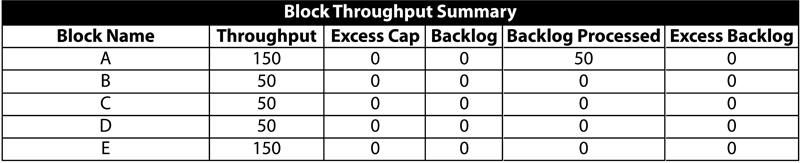A summary result table for the overall system for the simulation from 0 to 60 hours is shown in the following table.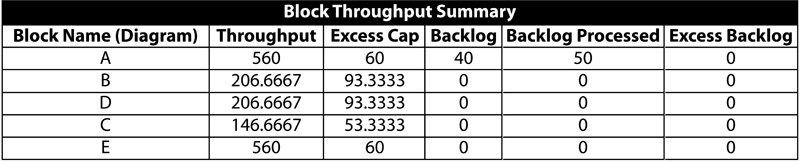### Variable Throughput Example

To examine variable throughput in phase diagrams, consider the phase diagram used above in the constant throughput example. All phase properties and linked RBDs remain unchanged for this illustration except that all the three phases now use variable throughput models. For the first phase, P1, the variable throughput model used is $\displaystyle y = x\,\!$ (which is the linear model $\displaystyle y = ax+ b\,\!$ with parameters $\displaystyle a = 1\,\!$ and $\displaystyle b = 0\,\!$ ). For the second phase, P2, the model used is $\displaystyle y = 3x\,\!$ (which is the linear model $\displaystyle y = ax+ b\,\!$ with parameters $\displaystyle a = 3\,\!$ and $\displaystyle b = 0\,\!$ ). The third phase uses $\displaystyle y = 0.5x\,\!$ as the variable throughput model (which is the linear model $\displaystyle y = ax + b\,\!$ with parameters $\displaystyle a = 0.5\,\!$ and $\displaystyle b = 0\,\!$ ). Phases P1 and P2 have a value of 500 units per hour set for the maximum throughput capacity. This relatively larger value of throughput is set for the two phases so that the phases do not reach their maximum throughput value during the simulation. Phase P3 has a value of 4 units per hour set as the maximum throughput capacity. As in the previous example, all phases have a duration of ten hours. For the sake of simplicity it is assumed that the blocks can never fail and that items are routed equally to each path. The ignore backlog option is left unchecked for all blocks in the phase diagram.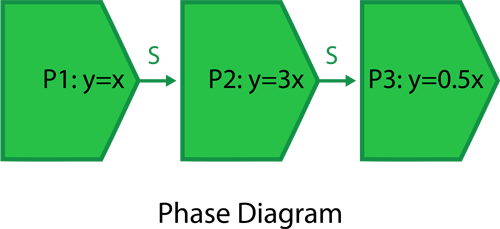If a throughput simulation with an end time of 60 hours is run on the phase diagram, the following scenario can be observed:

1. Phase P1 from 0 to 10 hours
a) The throughput from phase P1 follows a time-varying model given by $\displaystyle y = ax + b\,\!$ with parameters $\displaystyle a = 1\,\!$ and $\displaystyle b = 0\,\!$ . Thus, during the first hour the throughput sent by phase P1 to block A is:
\displaystyle \begin{align} Phase throughput from 0 to 1hr= & \frac{a}{2}(x_{2}^{2}-x_{1}^{2})+b({{x}_{2}}-{{x}_{1}}) \\ = & \frac{1}{2}({{1}^{2}}-{{0}^{2}})+0(1-0) \\ = & 0.5\text{ }units \end{align}\,\!

And the throughput sent by phase P1 to block A in the second hour is:
\displaystyle \begin{align} Phase throughput from 1 to 2 hrs= & \frac{a}{2}(x_{2}^{2}-x_{1}^{2})+b({{x}_{2}}-{{x}_{1}}) \\ = & \frac{1}{2}({{2}^{2}}-{{1}^{2}})+0(2-1) \\ = & 1.5\text{ }units \end{align}\,\!
It can be seen that the throughput from phase P1 sent to block A increases with time. But the capacity of block A remains constant at 10 units per hour during the entire phase P1. Thus, it becomes important to know the point of time when the number of units sent by P1 exceed the capacity of block A and the block starts accumulating a backlog. This can be obtained by solving the throughput model for phase P1 $\displaystyle (y = x)\,\!$ using the capacity of block A by substituting $\displaystyle y\,\!$ as the block capacity as shown next:
\displaystyle \begin{align} y= & x \\ or\text{ }10= & x \end{align}\,\!
Thus, at time $\displaystyle x = 10\,\!$ hours the number of units supplied per hour by phase P1 will equal the capacity of block A. At this point of time block A will function to its full capacity with no excess capacity and no backlog. Beyond this point the units sent by phase P1 will exceed the capacity of block A and the block will start accumulating a backlog. Since the duration of phase P1 is 10 hours, backlog at block A will not be a concern in this phase because up to this time the capacity of the block exceeds the units sent by phase P1. Thus, the total units sent by phase P1 to block A during the phase duration of 10 hours is:
\displaystyle \begin{align} = & \frac{a}{2}(x_{2}^{2}-x_{1}^{2})+b({{x}_{2}}-{{x}_{1}}) \\ = & \frac{1}{2}({{10}^{2}}-{{0}^{2}})+0(10-0) \\ = & 50\text{ }units \end{align}\,\!
The total number of units that can be processed by block A (or the capacity of A) during phase P1 is:
\displaystyle \begin{align} = & 10\times 10 \\ = & 100\text{ }units \end{align}\,\!
Thus, during the first phase, block A processes all 50 units sent by phase P1 with an excess capacity of 50 units (100-50=50 units).

b) The 50 units processed by block A from time 0 to 10 hours are routed equally over three paths to blocks B, C and D. Each of these blocks gets a total of 16.67 units from time 0 to 10 hours. The capacity of each of these blocks is 5 units per hour. Thus, the total capacity of each of the blocks from time 0 to 10 hours is 50 units. As a result, each of the three blocks processes 16.67 units in phase P1 with an excess capacity of 33.33 units.

c) Blocks B, C and D route each of their 16.67 units to block E. The capacity of E in this phase is 10 units per hour or 100 units for the ten hours. Thus, block E processes a total of 50 units from blocks B, C and D with an excess capacity of 50 units during the first phase P1. At 10 hours, phase P1 ends and phase P2 begins.

A summary result table for phase P1 is shown next.2. Phase P2 from 10 to 20 hours
a) The throughput of phase P2 follows the model $\displaystyle y = ax + b\,\!$ with $\displaystyle a = 3\,\!$ and $\displaystyle b = 0\,\!$ . The throughput from P2 goes to block A. The capacity of block A in this phase is 6 units per hour. The point of time when the number of units sent by P2 equals the capacity of block A can be obtained by substituting the capacity of block A into the model equation $\displaystyle (y = 3x)\,\!$ as shown next:
\displaystyle \begin{align} y= & 3x \\ or\text{ }6= & 3x \\ or\text{ }x= & 2 \end{align}\,\!
Thus, at $\displaystyle x = 2\,\!$ hours of phase P2 (or at the total simulation time of 12 hours), the number of units sent per hour by phase P2 equals the capacity of block A. Thus, during the first two hours of phase P2, block A will have excess capacity. The total number of units sent by phase P2 during the first two hours of the phase is:
\displaystyle \begin{align} = & \frac{a}{2}(x_{2}^{2}-x_{1}^{2})+b({{x}_{2}}-{{x}_{1}}) \\ = & \frac{3}{2}({{2}^{2}}-{{0}^{2}})+0(2-0) \\ = & 6\text{ }units \end{align}\,\!
The total capacity of block A during the first two hours of phase P2 is:
\displaystyle \begin{align} = & 6\times 2 \\ = & 12\text{ }units \end{align}\,\!
Thus block A processes a total of 6 units in the first two hours of phase P2 with an excess capacity of 6 units (12-6=6 units). During the last eight hours of the phase (from the second hour to the tenth hour) block A will have a backlog because the number of units sent by phase P2 will exceed block A's capacity. The total number of units sent by phase P2 during the last eight hours of the phase is:
\displaystyle \begin{align} = & \frac{a}{2}(x_{2}^{2}-x_{1}^{2})+b({{x}_{2}}-{{x}_{1}}) \\ = & \frac{3}{2}({{10}^{2}}-{{2}^{2}})+0(10-2) \\ = & 144\text{ }units \end{align}\,\!
The total capacity of block A during these eight hours of phase P2 is:
\displaystyle \begin{align} = & 6\times 8 \\ = & 48\text{ }units \end{align}\,\!
Thus, during the last eight hours of phase P2, block A will process a total of 48 units with a backlog of 96 units (144-48=96 units). A graphical representation of the above scenario for block A during phase P2 is shown in the figure below.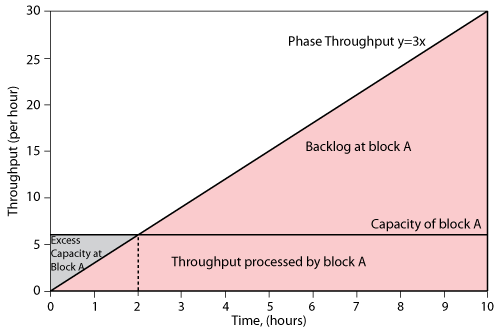b) The units processed by block A are routed equally to blocks B and D, as block C is absent in this phase. During the first two hours, block A sends a total of 6 units that are divided equally among blocks B and D, with each block getting 3 units. The capacity of these blocks in this phase is 5 units per hour. Thus the total capacity of each of these blocks during the first two hours of phase P2 is:
\displaystyle \begin{align} = & 5\times 2 \\ = & 10\text{ }units \end{align}\,\!
As a result, blocks B and D each process 3 units in the first two hours of phase P2 with an excess capacity of 7 units (10-3=7 units). During the last eight hours of phase P2, block A sends a total of 48 units to blocks B and D, with each block receiving 24 units. The capacity of each of these blocks during these eight hours is:
\displaystyle \begin{align} = & 5\times 8 \\ = & 40\text{ }units \end{align}\,\!
Thus, B and D process 24 units in the last eight hours of phase P2, with an excess capacity of 16 units (40-24=16 units).

c) The units processed by blocks B and D are sent to block E. The capacity of E in this phase is 6 units per hour. During the first two hours of phase P2, a total of 6 units are sent to block E. The total capacity of block E during this period of time is:
\displaystyle \begin{align} = & 6\times 2 \\ = & 12\text{ }units \end{align}\,\!
Consequently block E processes 6 units in the first two hours of phase P2 with an excess capacity of 6 units (12-6=6 units). During the last eight hours of the phase, blocks B and D send a total of 48 units to block E. The capacity of block E during this time is:
\displaystyle \begin{align} = & 6\times 8 \\ = & 48\text{ }units \end{align}\,\!
Thus, block E processes 48 units in the last eight hours of P2 with no excess capacity and no backlog. At 20 hrs phase P2 ends and phase P3 begins.

A summary result table for phase P2 is shown next.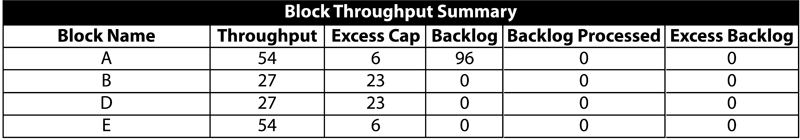3. Phase P3 from 20 to 30 hours
a) The throughput of phase P3 follows the model $\displaystyle y = ax + b\,\!$ with $\displaystyle a = 0.5\,\!$ and $\displaystyle b = 0\,\!$ . A maximum throughput capacity of 4 units per hour is also set for this phase. The throughput from this phase goes to block A. The capacity of block A in this phase is 15 units per hour. Thus, it can be seen that the throughput sent by phase P3 will never exceed the capacity of block A in this phase. It can also be seen that at some point of time the throughput of phase P3 will reach the maximum throughput capacity of 4 units per hour and, thereafter, a constant throughput of 4 units per hour will be sent by phase P3. This point of time can be calculated by solving the linear throughput model for the phase $\displaystyle y = 0.5x\,\!$ by substituting $\displaystyle y\,\!$ as the maximum throughput capacity as shown next:
\displaystyle \begin{align} y= & 0.5x \\ or\text{ }4= & 0.5x \\ or\text{ }x= & 8 \end{align}\,\!
Thus, at time $\displaystyle x = 8\,\!$ hours of phase P3 (or at the time of 28 hours) the number of units sent per hour by phase P3 will reach the constant value of 4 units per hour. In the first eight hours of the phase the throughput will remain variable. The total number of units sent by P3 to block A during this time is given by:
\displaystyle \begin{align} = & \frac{a}{2}(x_{2}^{2}-x_{1}^{2})+b({{x}_{2}}-{{x}_{1}}) \\ = & \frac{0.5}{2}({{8}^{2}}-{{0}^{2}})+0(8-0) \\ = & 16\text{ }units \end{align}\,\!
The total capacity of block A during the first eight hours of phase P3 is:
\displaystyle \begin{align} = & 15\times 8 \\ = & 120\text{ }units \end{align}\,\!
Consequently, it can be seen that block A has an excess capacity of 104 units (120-16=104 units). Thus, block A will be able to process all of the 96 units of backlog from phase P2 during these eight hours. As a result, block A will process a total of 112 units (16 units from P3 and 96 units from the backlog of phase P2) with an excess capacity of 8 units (120-112=8 units) during the first eight hours of phase P3. In the last two hours of this phase, a constant throughput of 4 units per hour is sent to block A, thus the total number of units sent to the block during the last two hours are:
\displaystyle \begin{align} = & 4\times 2 \\ = & 8\text{ }units \end{align}\,\!
The capacity of block A during these two hours is:
\displaystyle \begin{align} = & 15\times 2 \\ = & 30\text{ }units \end{align}\,\!
Thus, block A will process 8 units in the last two hours of phase P3 with an excess capacity of 22 units (30-8=22 units).

b) The units processed by block A are routed equally over three paths to blocks B, C and D, as block C is available again in this phase. During the first eight hours of phase P3 a total of 112 units are sent to blocks B, C and D, with each block receiving 37.33 units. The capacity of each of these block is 5 units per hour, thus the total capacity of each of the blocks during the first eight hours is:
\displaystyle \begin{align} = & 5\times 8 \\ = & 40\text{ }units \end{align}\,\!
As a result, blocks B, C and D each process 37.33 units in the first eight hour of phase P3 with an excess capacity of 2.67 units (40-37.33=2.67 units). In the last two hours of the phase, block A sends a total of 8 units, with each block getting 2.67 units. The capacity of blocks B, C or D during these two hours is:
\displaystyle \begin{align} = & 5\times 2 \\ = & 10\text{ }units \end{align}\,\!
Thus, blocks B, C and D each process 2.67 units in the last two hours of phase P3 with an excess capacity of 7.33 units (10-2.67=7.33 units). At the end of these two hours phase P3 gets completed. This also marks the end of the simulation.
A summary result table for phase P3 is shown next.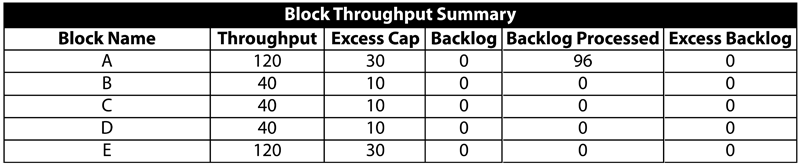A summary result table for the overall system for the simulation from 0 to 30 hrs is shown in the following table.